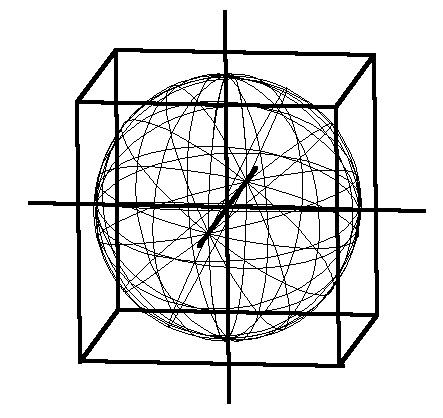# Cubing the Sphere

Similar to Squaring the Circle but in 3D. Used for calculating volume changes of spheres such as may be needed using Square Law and Inverse Square Law computing expanding and contracting sound waves about a creative source.Volume decreases with increased potential.

Volume increases with decreased potential.

Cube Root of the Volume = SqRt of the Area = Length

"Cubing the Sphere" (Sphere contiguous with and enclosed by a Cube)

Sphere Volume = 2 X 315 X 5153 = 147,879,835,542

Cube Volume = 65613 = 282,429,536,481

Ratio: 282,429,536,481:147,879,835,542::19,683:10,306 = Diminished Octave or Seventh.

Sphere to Cube = Minor Seventh = 9:5::(2 X 315 X 5153):(65613)

Square-Cube Law
The square-cube law (or cube-square law) is a principle, drawn from the mathematics of proportion, that is applied in engineering and biomechanics. It was first demonstrated in 1638 in Galileo's Two New Sciences. It states:

"When an object undergoes a proportional increase in size, its new volume is proportional to the cube of the multiplier and its new surface area is proportional to the square of the multiplier."